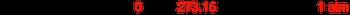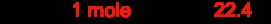## GOB Chemistry

Learn the toughest concepts covered in your GOB - General, Organic, and Biological Chemistry class with step-by-step video tutorials and practice problems.

Gases, Liquids and Solids

# Standard Temperature and Pressure

In order to accurately study the effect that changes in pressure, temperature and moles have on volume, chemists will often run their experiments under Standard Temperature and Pressure conditions

1
concept

## Standard Temperature and Pressure33s
Play a video:
standard temperature and pressure. Abbreviated as STP is a commonly used term in calculations involving gas, is now we're going to say at STP the temperature is measured as either zero degrees Celsius or 273. Kelvin. Now we know that when we're dealing with gas calculations, we tend to go with Kelvin. Now the pressure at STP is one atmosphere. So just remember, when you hear STP, it means 273.15 Kelvin and for pressure, one atmosphere.2
example

## Standard Temperature and Pressure Example 12m
Play a video:
3
concept

## Standard Temperature and Pressure1m
Play a video:
with the idea of STP were faced with a new idea the standard molar volume. Now we're going to say that it represents the volume of one mole of an ideal gas at STP. Alright, so here we're gonna say volume equals moles. Times are t overpay. We're dealing with one mole of the gas Are is just are constant And we're gonna say we're dealing with STP. So our pressure will be to 73.15 Kelvin and our pressure will just simply be one atmosphere we see here that the moles cancel out Kelvin's cancel out atmospheres cancel out. So here we'll have our volume and leaders. When we plug this in, we get 22.4 leaders. This would represent our standard molar volume for one mole of gas and ideal gas. Now this helps to establish a relationship between moles and volumes. And because we have a relationship between Moland volumes, we can create a new conversion factor. And that conversion factor would be that for any one mole of an ideal gas at STP, it's Moeller volume would be 22 4 leaders. So just remember, if we're dealing with STP and we're dealing with one mole of any gas, then it's standard molar. Volume will be 22.4 leaders4
example

## Standard Temperature and Pressure Example 21m
Play a video:
5
Problem

A sample of dichloromethane gas (CH2Cl2) occupies 32.6 L at 310 K and 5.30 atm. Determine its volume at STP?

6
Problem

Which gas sample has the greatest volume at STP?

7
Problem

Nitrogen and hydrogen combine to form ammonia via the following reaction:

1 N2 (s)  +  3 H2 (g) → 2 NH3 (g)

What mass of nitrogen is required to completely react with 800.0 mL Hat STP?